# RD Sharma Solutions for Class 8 Maths Chapter 22 Mensuration - III (Surface Area and Volume of a Right Circular Cylinder) Exercise 22.2

In the previous section, we studied about the surface area of a hollow cylinder, in Exercise 22.2, we shall discuss problems based on the volume of a hollow cylinder (solids like iron pipes, rubber tubes etc are examples of hollow cylinders). For a quick reference, solutions of this exercise can be accessed at RD Sharma Class 8 Solutions which are formulated by our experts in a simple and understandable language. Students can also download the RD Sharma Class 8 pdf from the links available below.

## Download the Pdf of RD Sharma Solutions for Class 8 Maths Exercise 22.2 Chapter 22 Mensuration – III (Surface Area and Volume of a Right Circular Cylinder)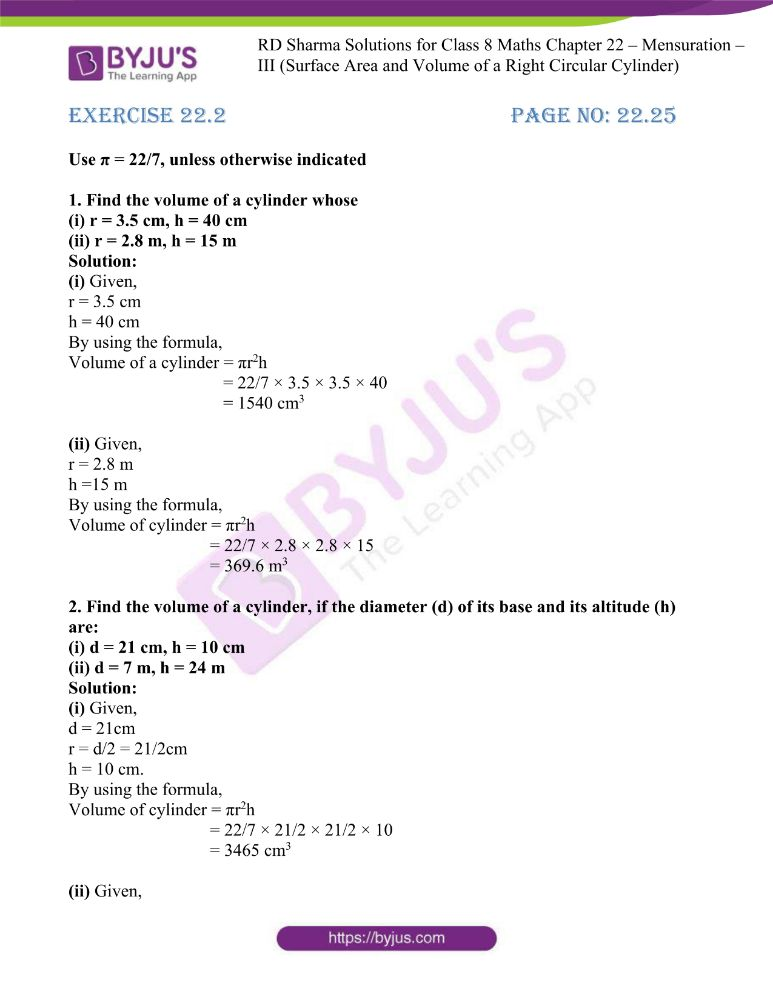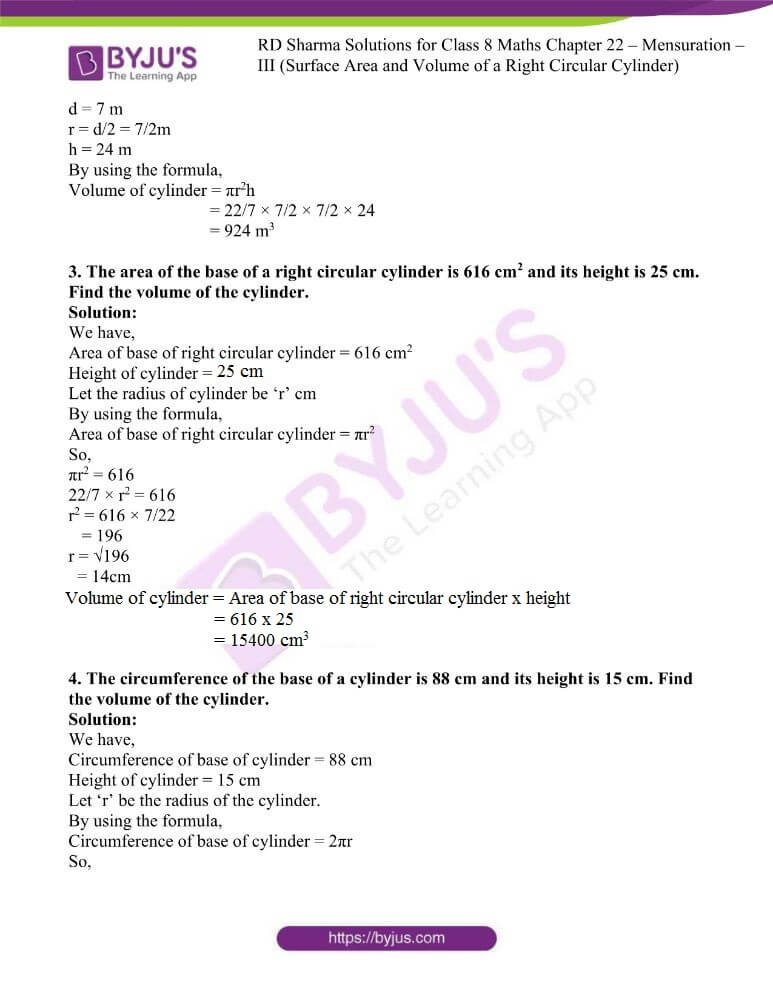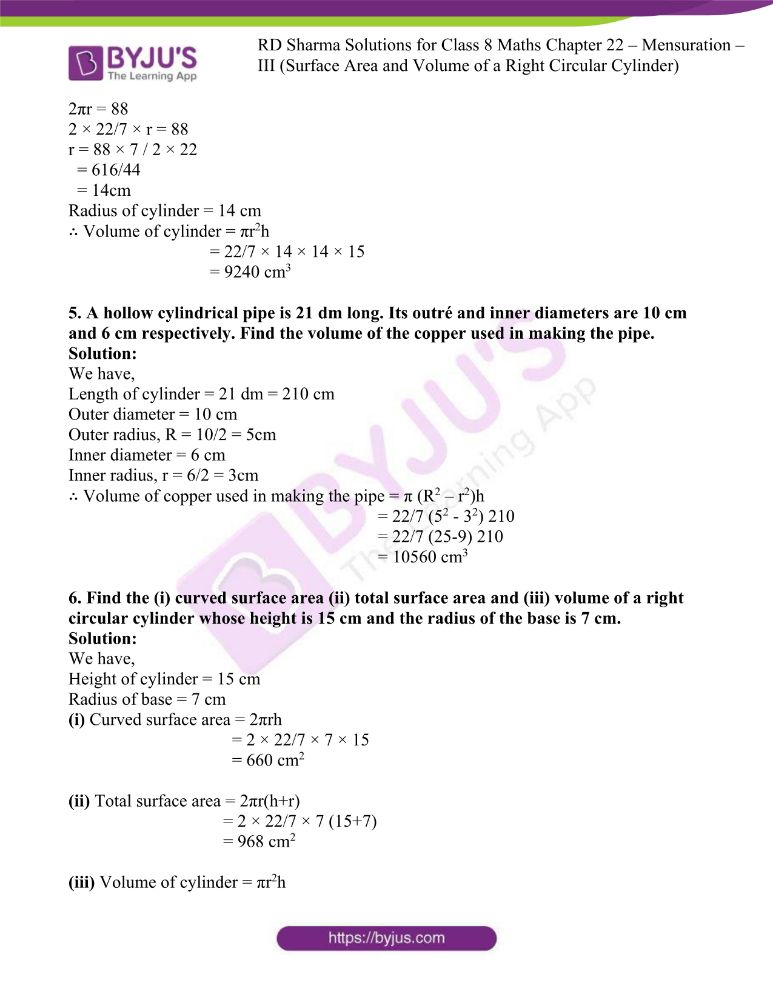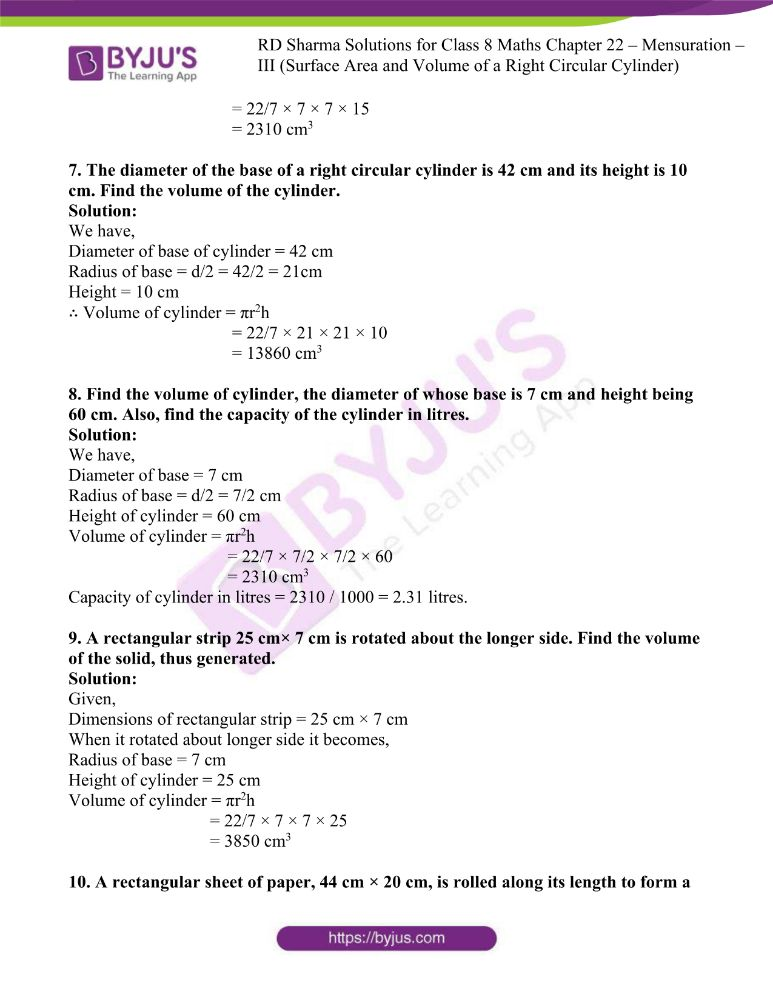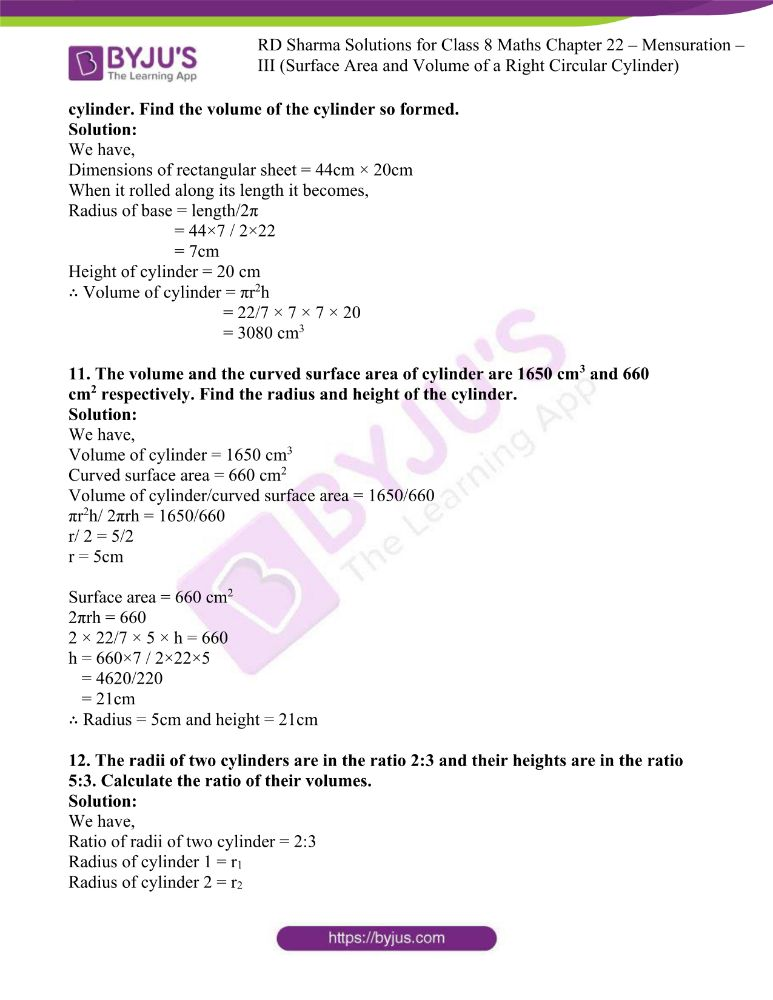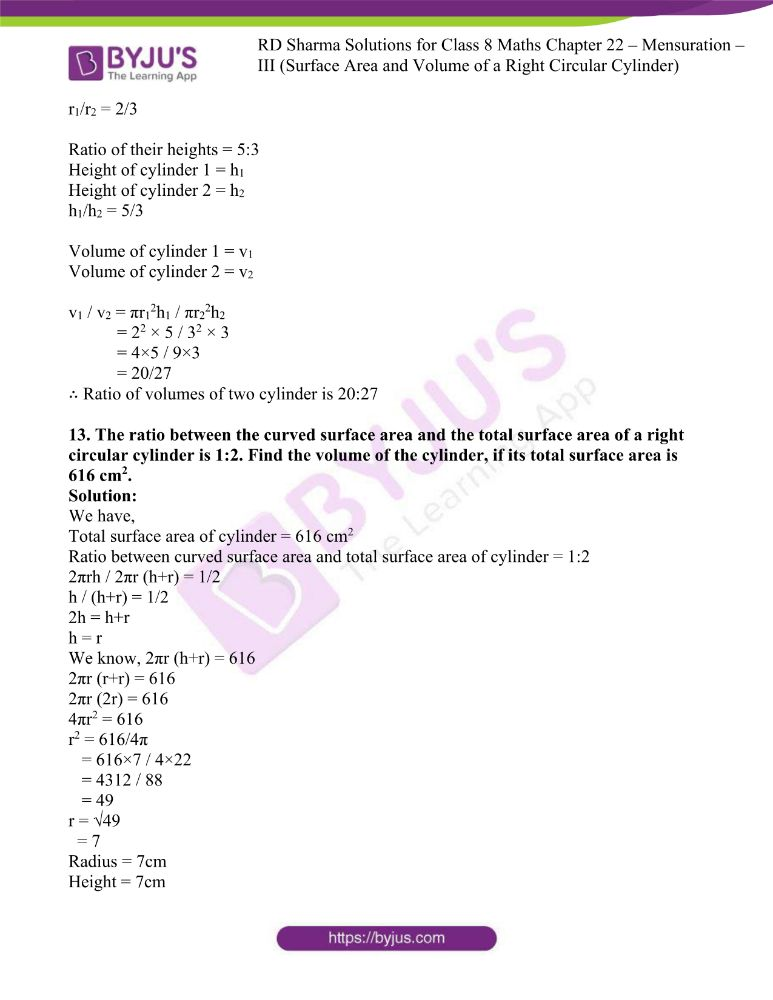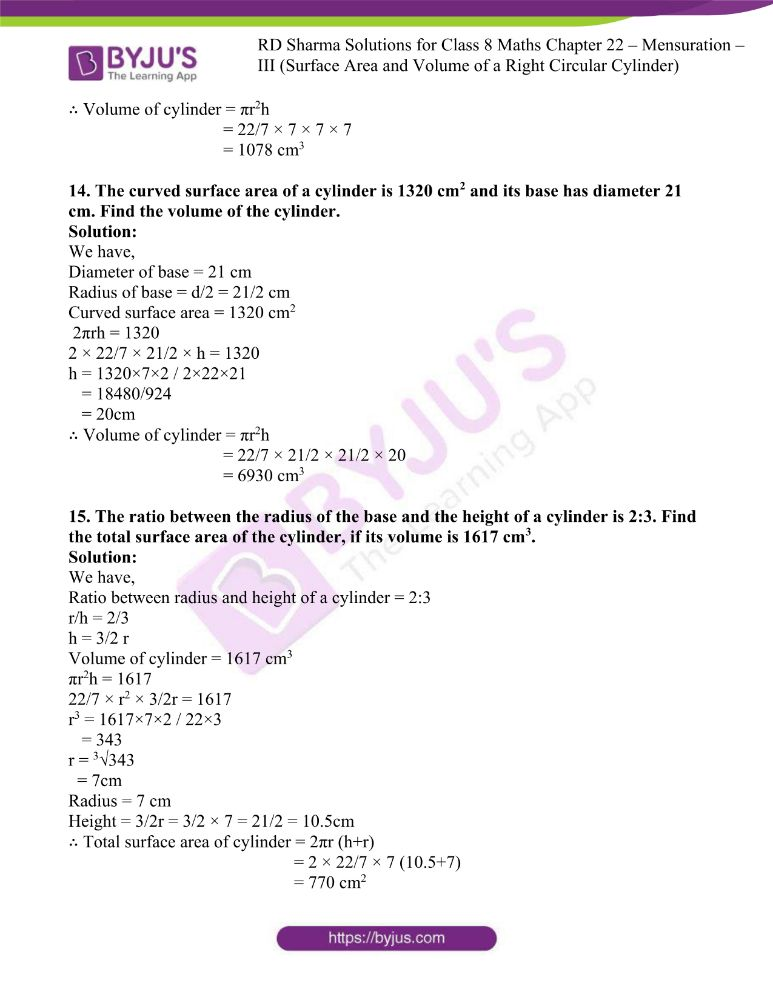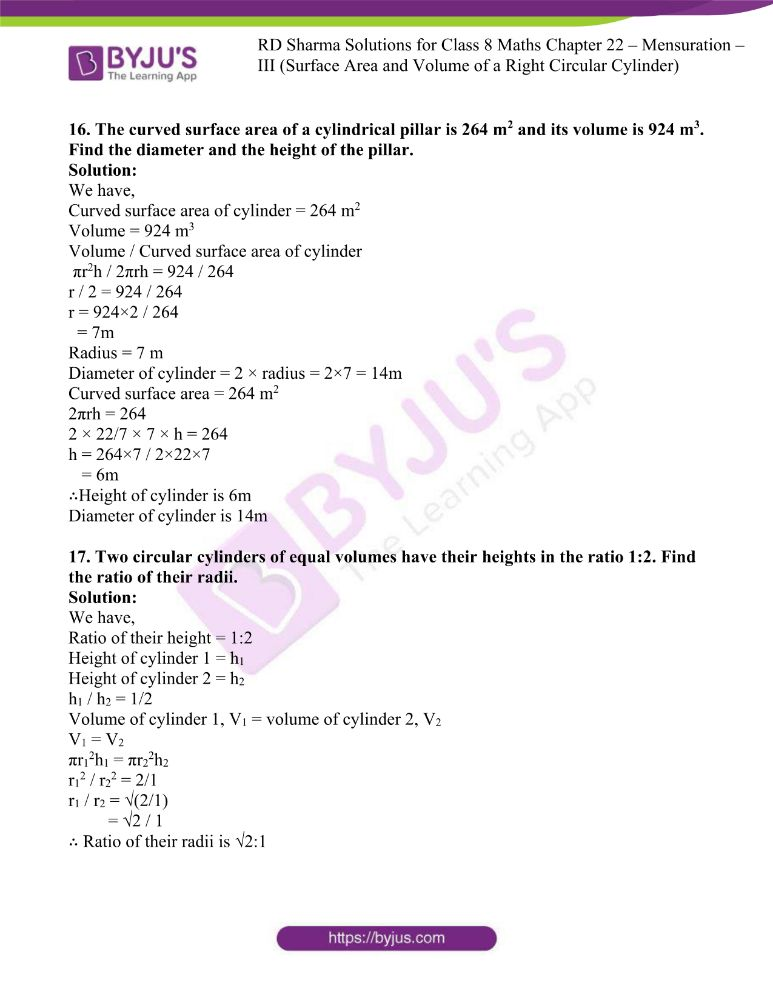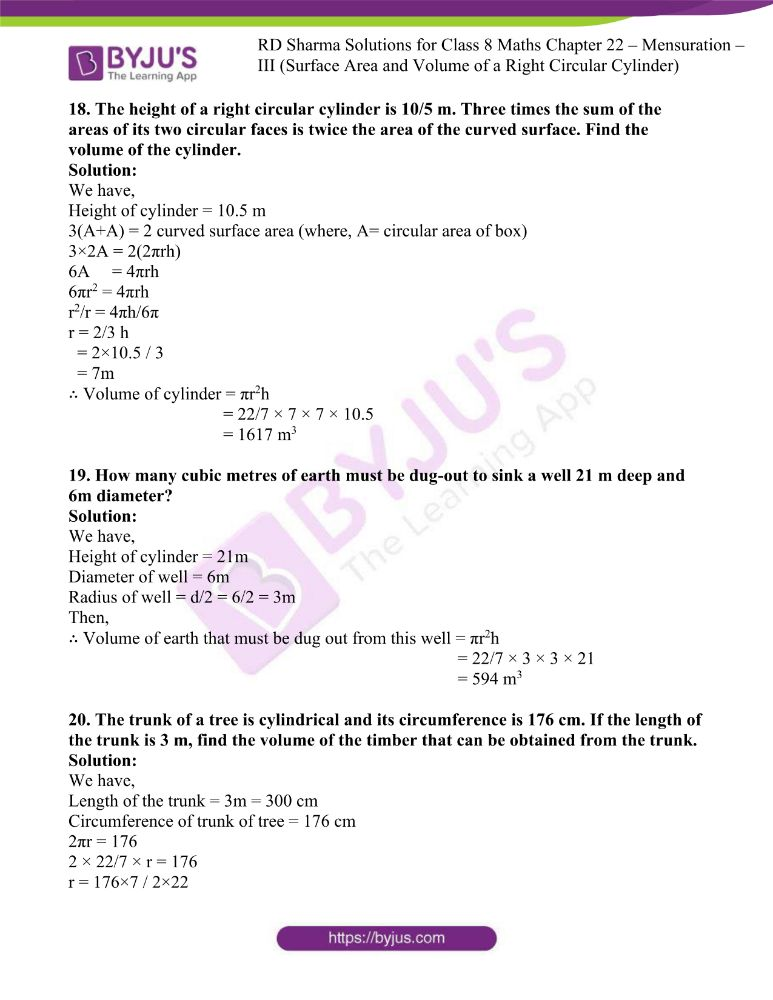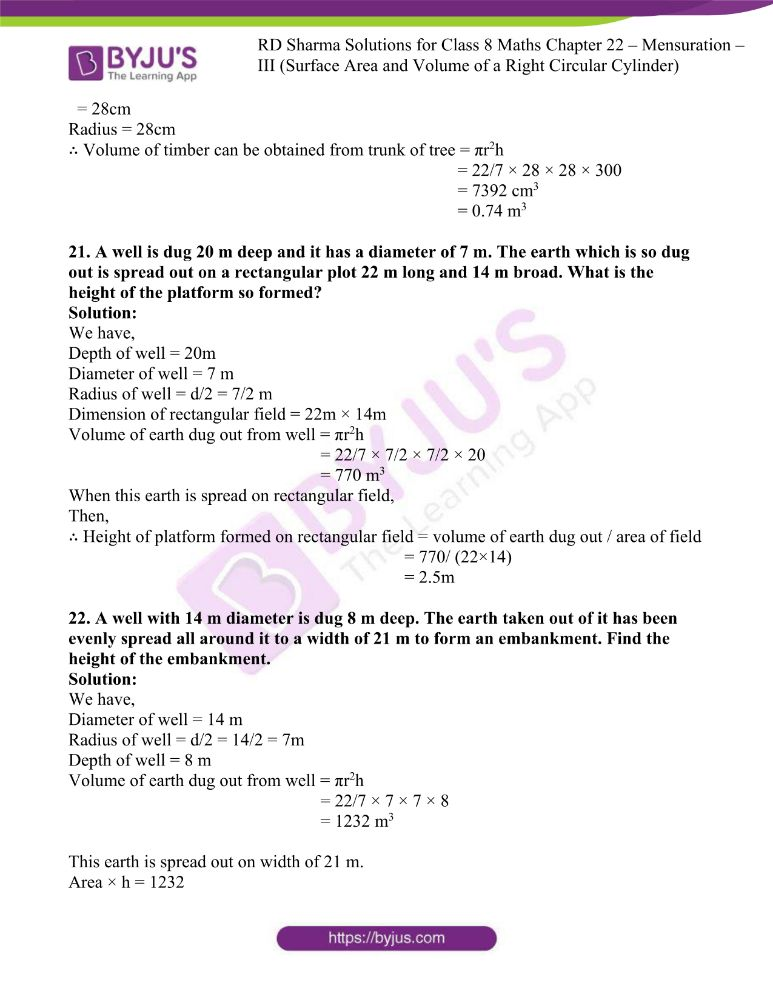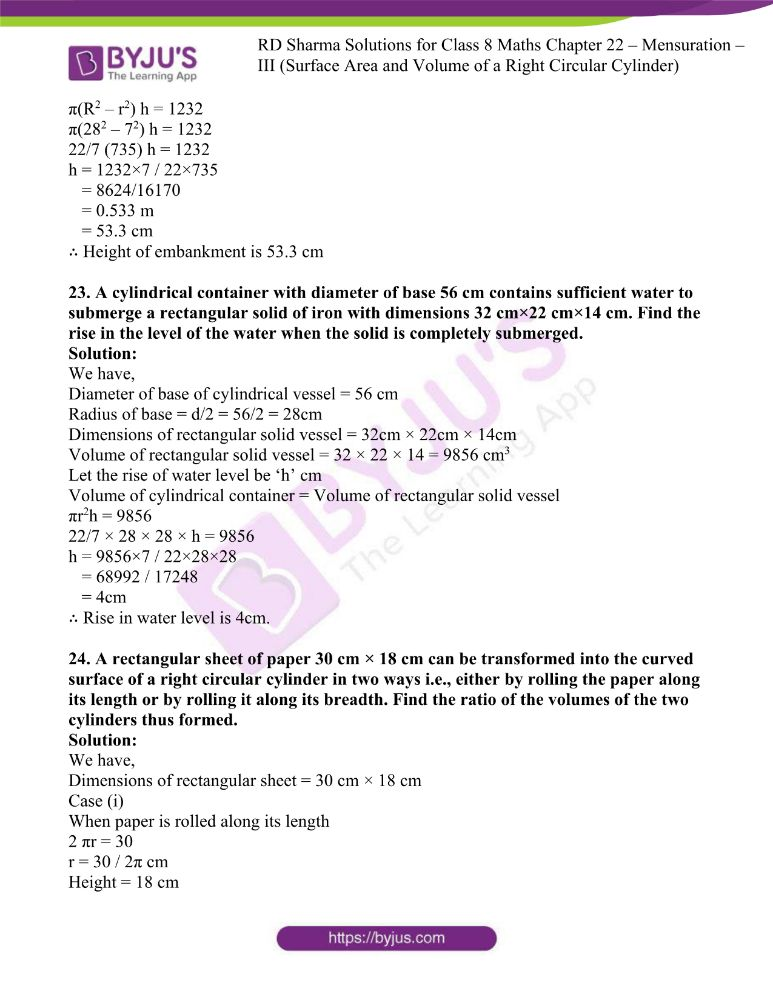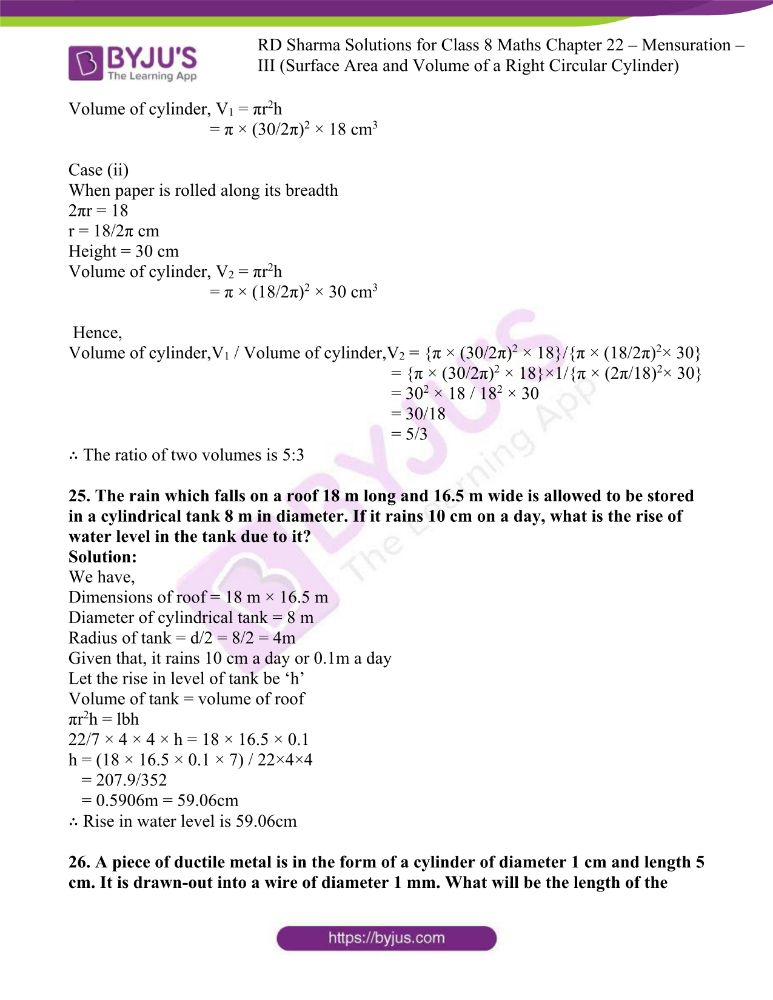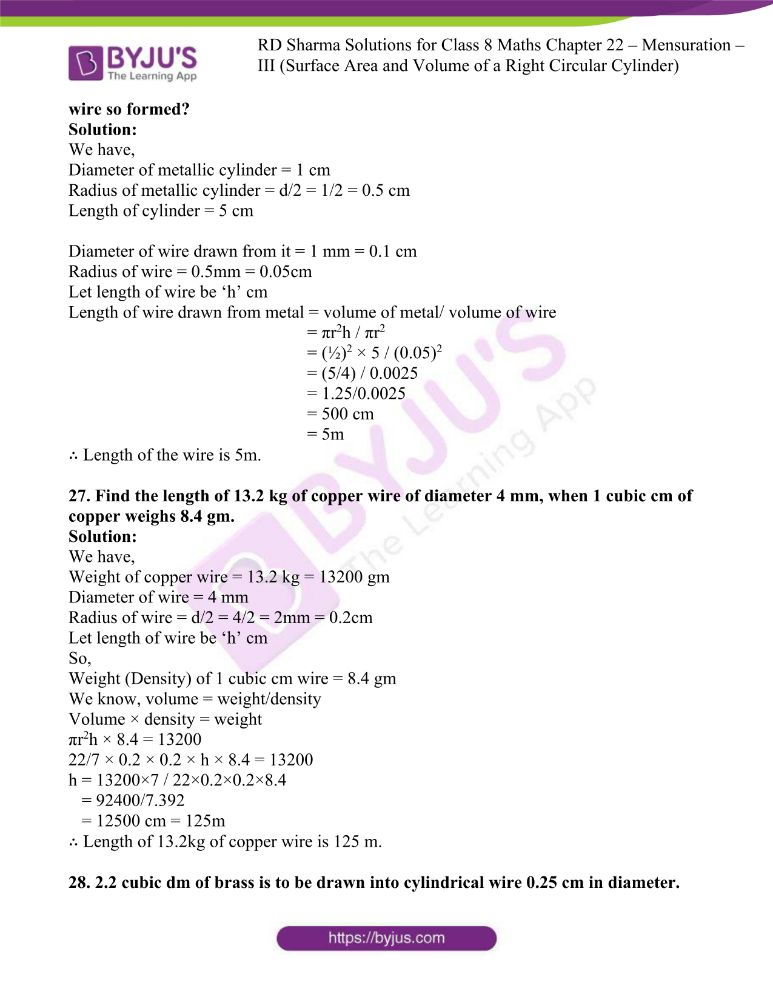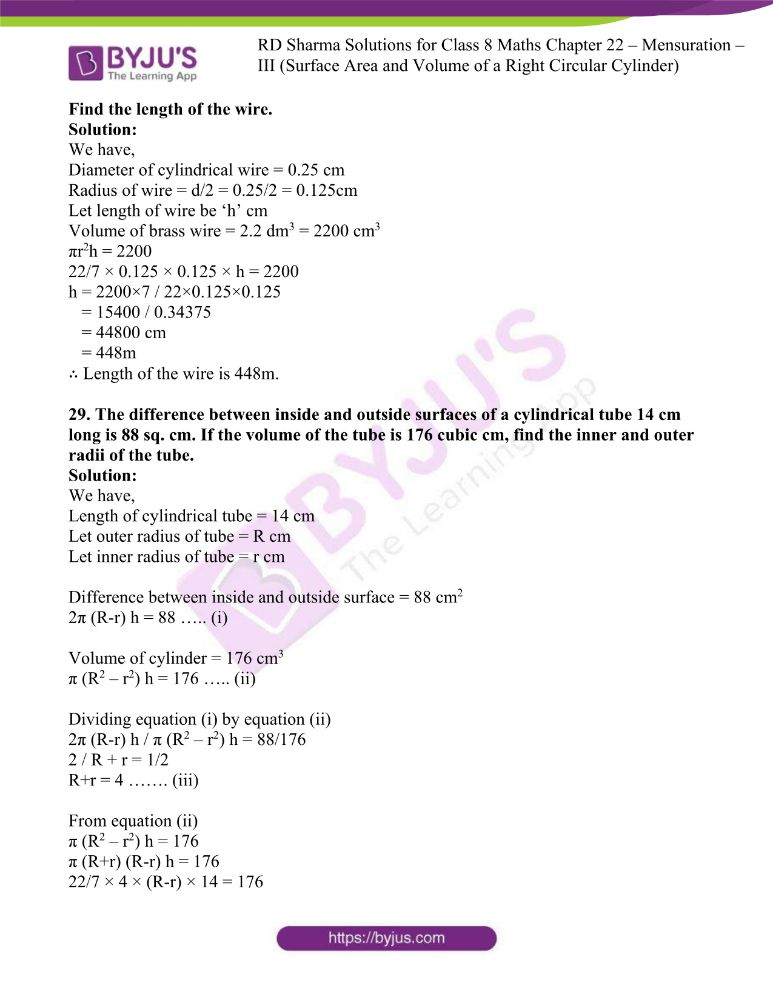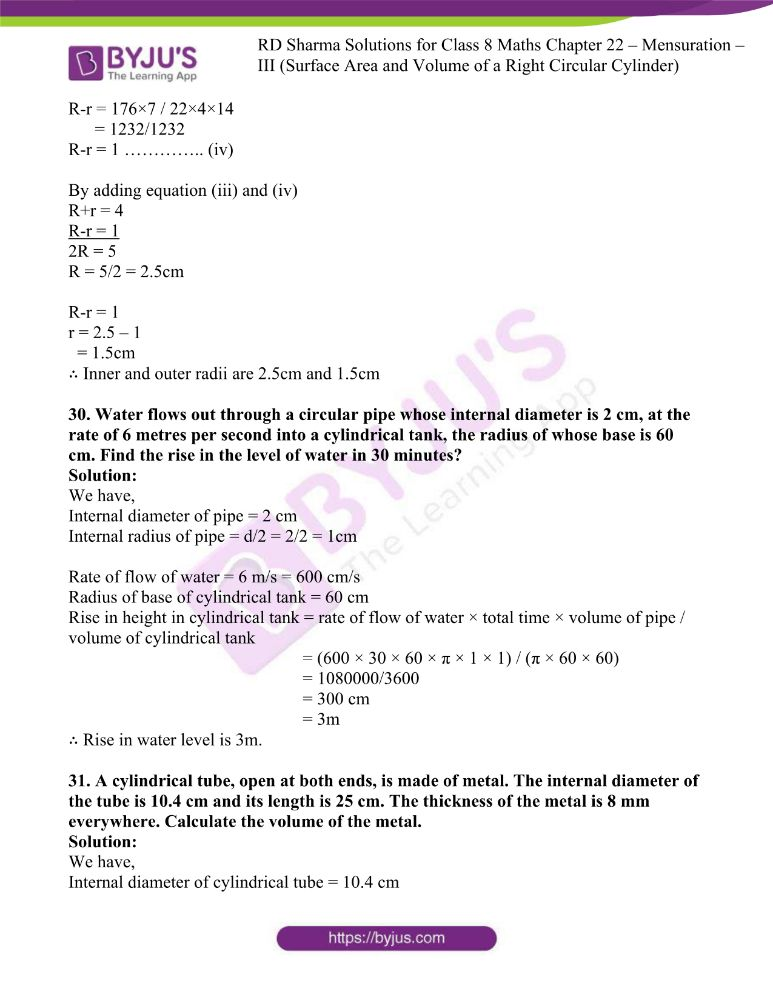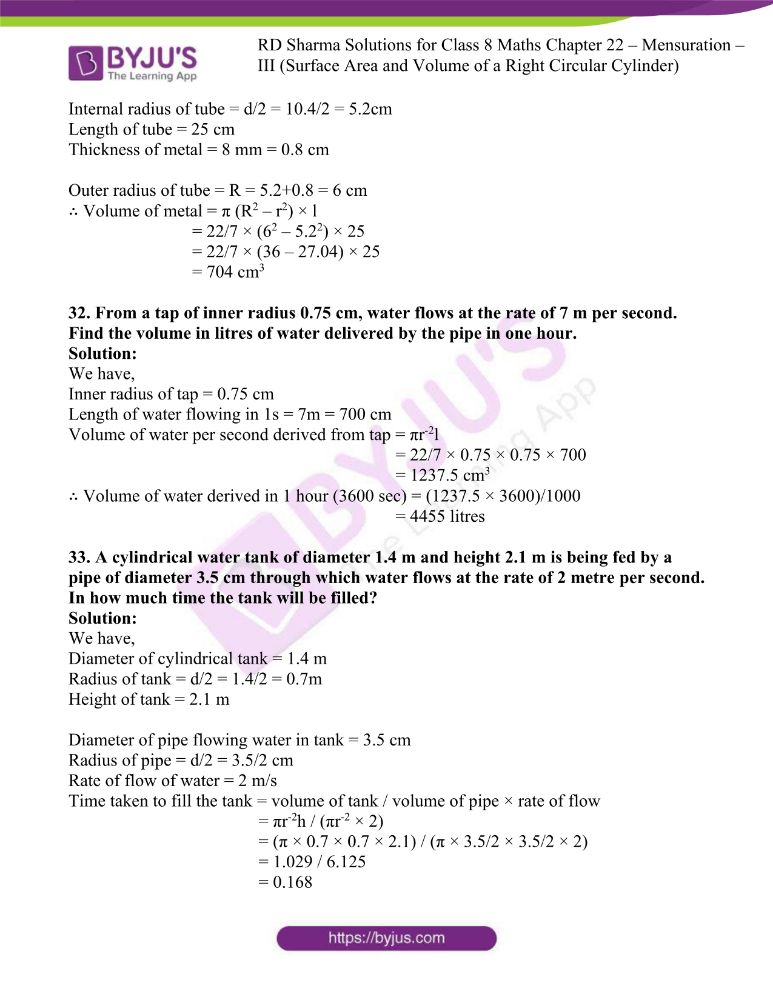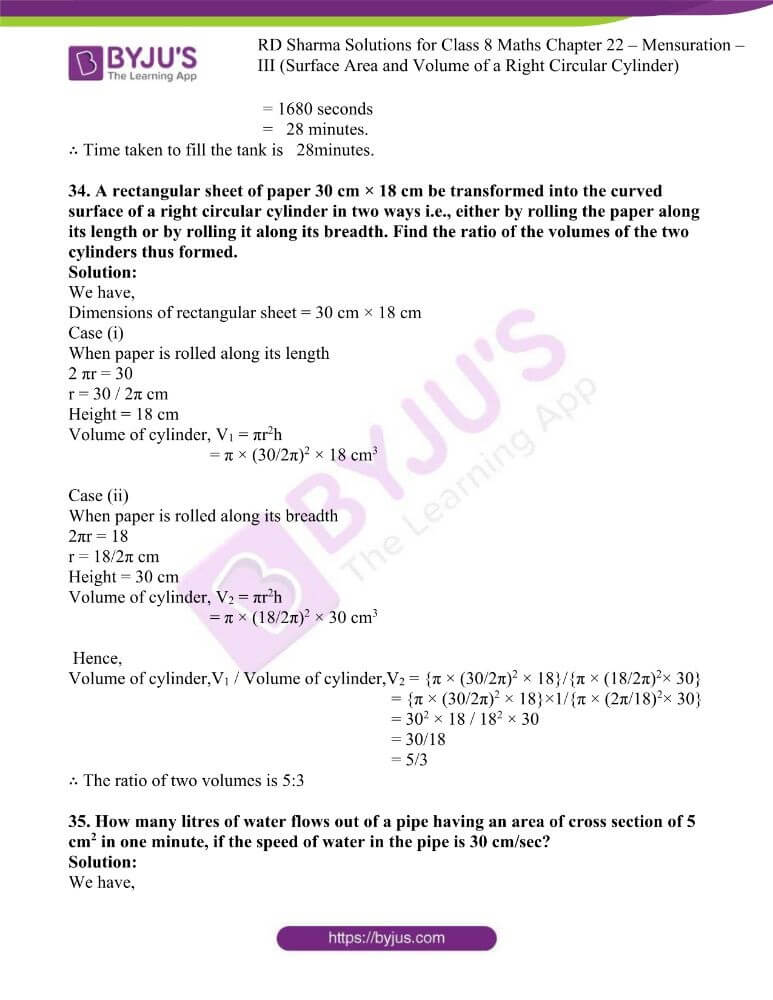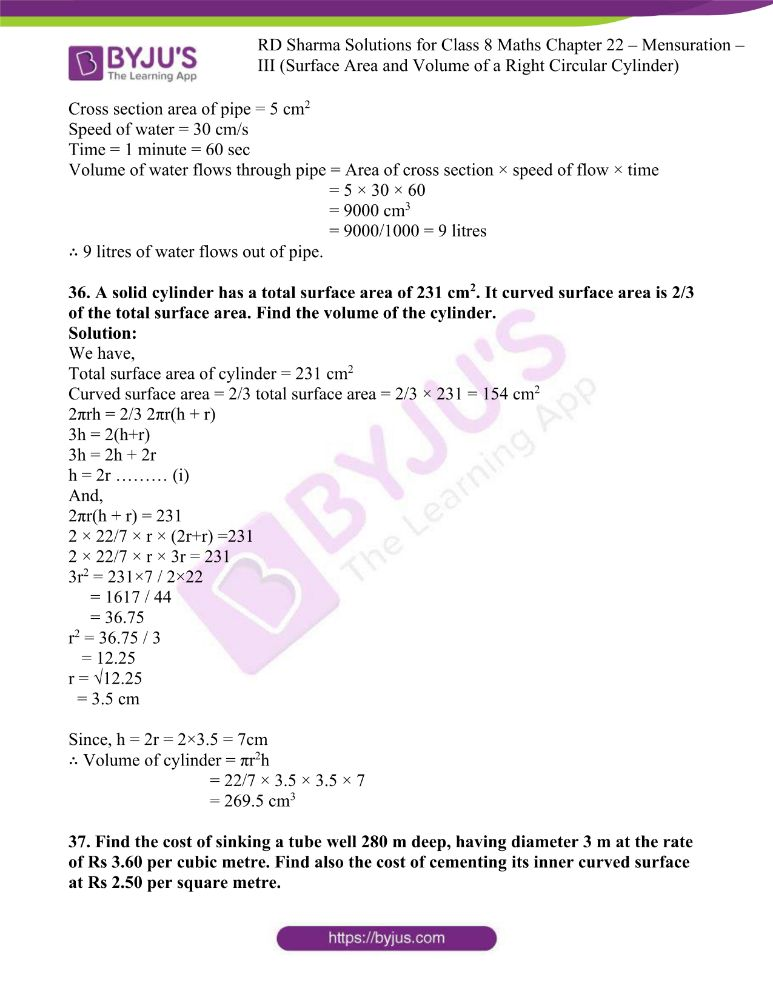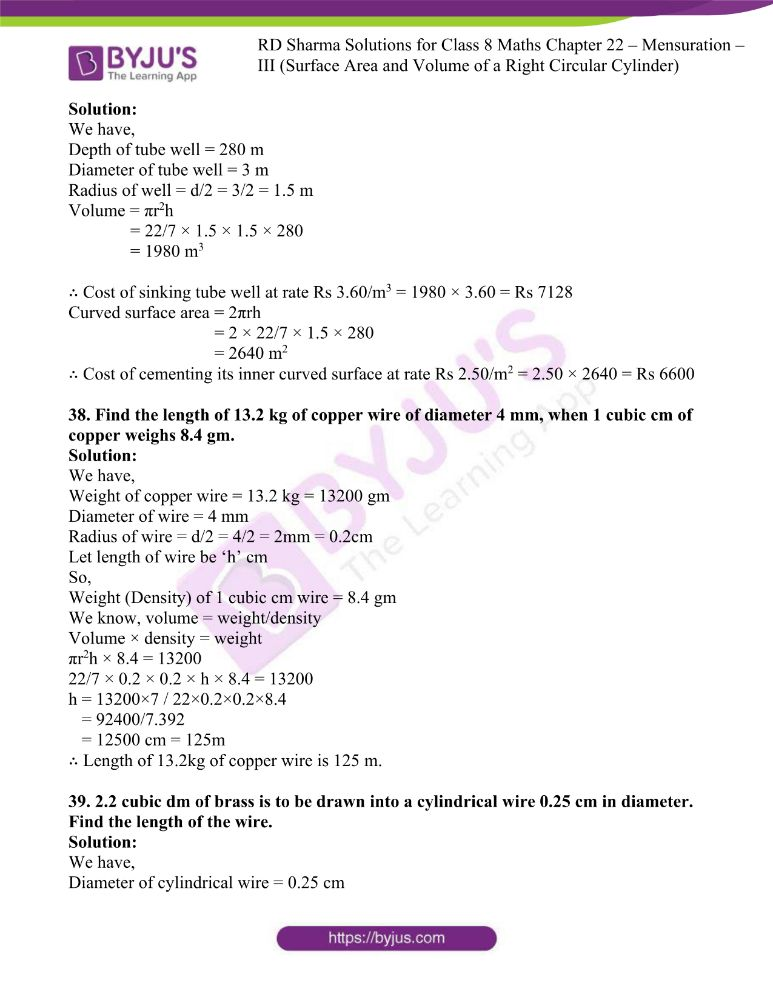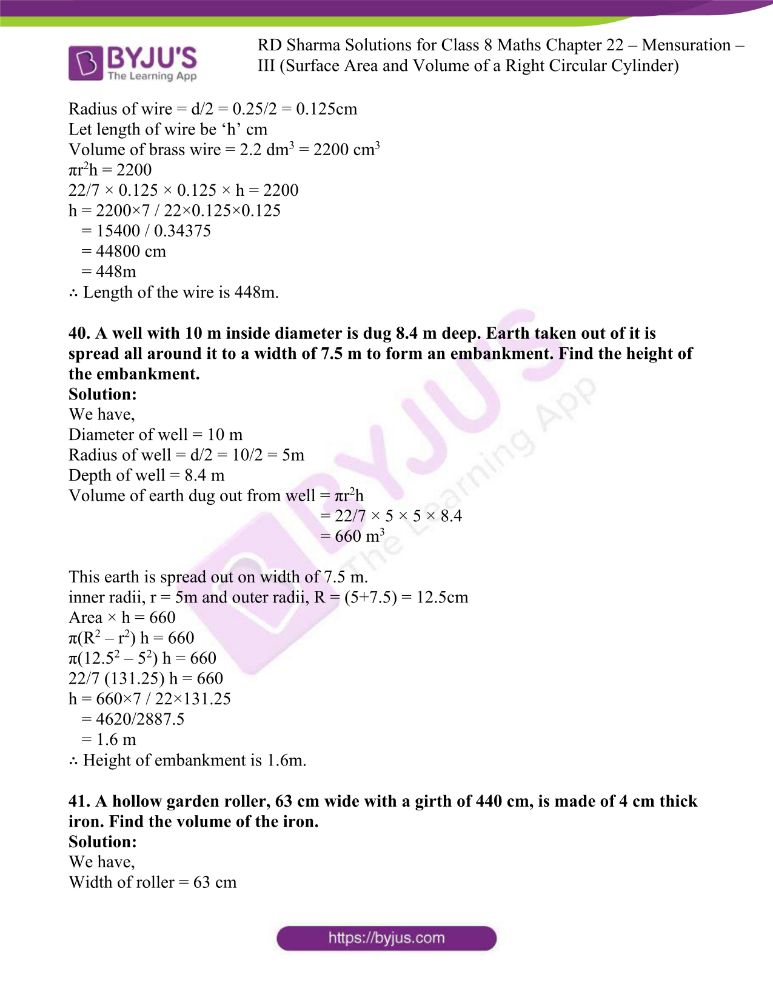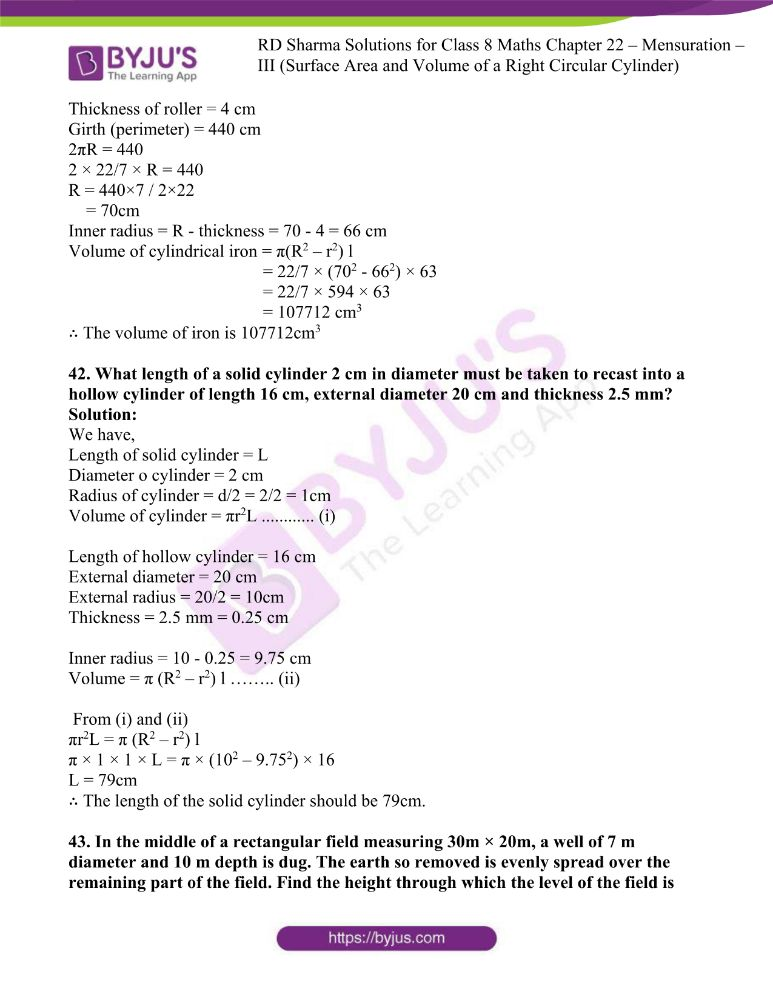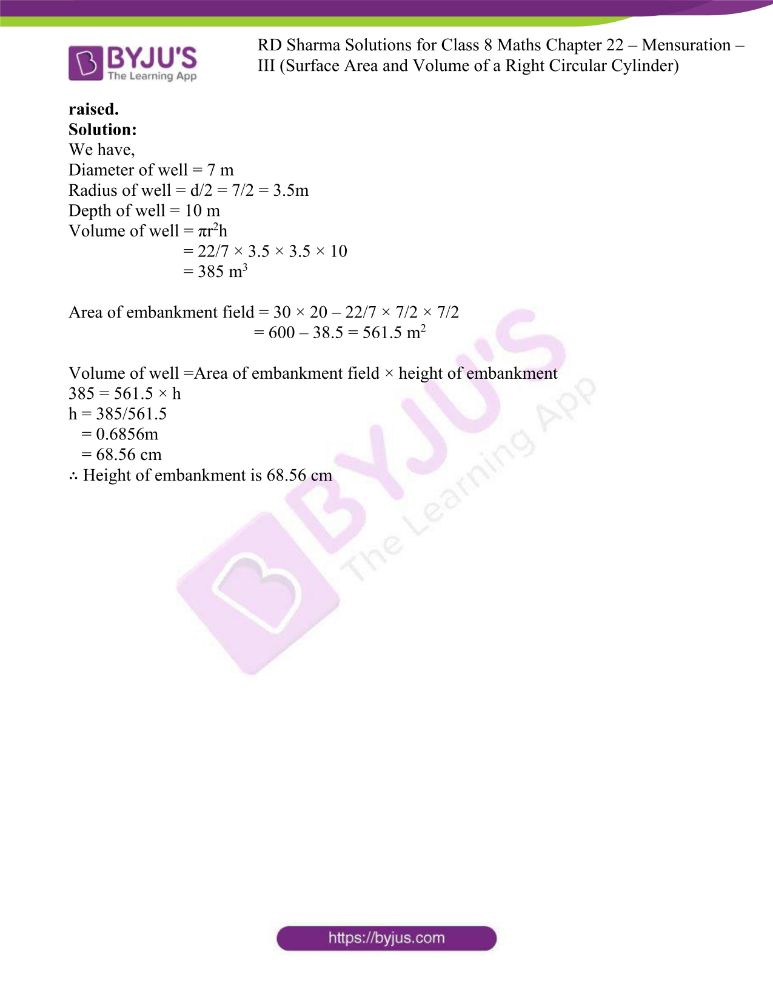### Access another exercise of RD Sharma Class 8 Maths Solutions Chapter 22 Mensuration – III (Surface Area and Volume of a Right Circular Cylinder)

Exercise 22.1 Solutions

### Access answers to RD Sharma Maths Solutions For Class 8 Exercise 22.2 Chapter 22 Mensuration – II (Volumes and Surface Areas of a Cuboid and a Cube)

Use π = 22/7, unless otherwise indicated

1. Find the volume of a cylinder whose
(i) r = 3.5 cm, h = 40 cm
(ii) r = 2.8 m, h = 15 m

Solution:

(i) Given,

r = 3.5 cm

h = 40 cm

By using the formula,

Volume of a cylinder = πr2h

= 22/7 × 3.5 × 3.5 × 40

= 1540 cm3

(ii) Given,

r = 2.8 m

h =15 m

By using the formula,

Volume of cylinder = πr2h

= 22/7 × 2.8 × 2.8 × 15

= 369.6 m3

2. Find the volume of a cylinder, if the diameter (d) of its base and its altitude (h) are:
(i) d = 21 cm, h = 10 cm
(ii) d = 7 m, h = 24 m

Solution:

(i) Given,

d = 21cm

r = d/2 = 21/2cm

h = 10 cm.

By using the formula,

Volume of cylinder = πr2h

= 22/7 × 21/2 × 21/2 × 10

= 3465 cm3

(ii) Given,

d = 7 m

r = d/2 = 7/2m

h = 24 m

By using the formula,

Volume of cylinder = πr2h

= 22/7 × 7/2 × 7/2 × 24

= 924 m3

3. The area of the base of a right circular cylinder is 616 cm2 and its height is 25 cm. Find the volume of the cylinder.

Solution:

We have,

Area of base of right circular cylinder = 616 cm2

Height of cylinder = 25 cm

Let the radius of cylinder be ‘r’ cm

By using the formula,

Area of base of right circular cylinder = πr2

So,

πr2 = 616

22/7 × r2 = 616

r2 = 616 × 7/22

= 196

r = √196

= 14cm

Volume of cylinder = Area of base of right circular cylinder × height

= 616 × 25

= 15400 cm3

4. The circumference of the base of a cylinder is 88 cm and its height is 15 cm. Find the volume of the cylinder.

Solution:

We have,

Circumference of base of cylinder = 88 cm

Height of cylinder = 15 cm

Let ‘r’ be the radius of the cylinder.

By using the formula,

Circumference of base of cylinder = 2πr

So,

2πr = 88

2 × 22/7 × r = 88

r = 88 × 7 / 2 × 22

= 616/44

= 14cm

Radius of cylinder = 14 cm

∴ Volume of cylinder = πr2h

= 22/7 × 14 × 14 × 15

= 9240 cm3

5. A hollow cylindrical pipe is 21 dm long. Its outré and inner diameters are 10 cm and 6 cm respectively. Find the volume of the copper used in making the pipe.

Solution:

We have,

Length of cylinder = 21 dm = 210 cm

Outer diameter = 10 cm

Outer radius, R = 10/2 = 5cm

Inner diameter = 6 cm

Inner radius, r = 6/2 = 3cm

∴ Volume of copper used in making the pipe = π (R2 – r2)h

= 22/7 (52 – 32) 210

= 22/7 (25-9) 210

= 10560 cm3

6. Find the (i) curved surface area (ii) total surface area and (iii) volume of a right circular cylinder whose height is 15 cm and the radius of the base is 7 cm.

Solution:

We have,

Height of cylinder = 15 cm

Radius of base = 7 cm

(i) Curved surface area = 2πrh

= 2 × 22/7 × 7 × 15

= 660 cm2

(ii) Total surface area = 2πr(h+r)

= 2 × 22/7 × 7 (15+7)

= 968 cm2

(iii) Volume of cylinder = πr2h

= 22/7 × 7 × 7 × 15

= 2310 cm3

7. The diameter of the base of a right circular cylinder is 42 cm and its height is 10 cm. Find the volume of the cylinder.

Solution:

We have,

Diameter of base of cylinder = 42 cm

Radius of base = d/2 = 42/2 = 21cm

Height = 10 cm

∴ Volume of cylinder = πr2h

= 22/7 × 21 × 21 × 10

= 13860 cm3

8. Find the volume of cylinder, the diameter of whose base is 7 cm and height being 60 cm. Also, find the capacity of the cylinder in litres.

Solution:

We have,

Diameter of base = 7 cm

Radius of base = d/2 = 7/2 cm

Height of cylinder = 60 cm

Volume of cylinder = πr2h

= 22/7 × 7/2 × 7/2 × 60

= 2310 cm3

Capacity of cylinder in litres = 2310 / 1000 = 2.31 litres.

9. A rectangular strip 25 cm× 7 cm is rotated about the longer side. Find the volume of the solid, thus generated.

Solution:

Given,

Dimensions of rectangular strip = 25 cm × 7 cm

When it rotated about longer side it becomes,

Radius of base = 7 cm

Height of cylinder = 25 cm

Volume of cylinder = πr2h

= 22/7 × 7 × 7 × 25

= 3850 cm3

10. A rectangular sheet of paper, 44 cm × 20 cm, is rolled along its length to form a cylinder. Find the volume of the cylinder so formed.

Solution:

We have,

Dimensions of rectangular sheet = 44cm × 20cm

When it rolled along its length it becomes,

= 44×7 / 2×22

= 7cm

Height of cylinder = 20 cm

∴ Volume of cylinder = πr2h

= 22/7 × 7 × 7 × 20

= 3080 cm3

11. The volume and the curved surface area of cylinder are 1650 cm3 and 660 cm2 respectively. Find the radius and height of the cylinder.

Solution:

We have,

Volume of cylinder = 1650 cm3

Curved surface area = 660 cm2

Volume of cylinder/curved surface area = 1650/660

πr2h/ 2πrh = 1650/660

r/ 2 = 5/2

r = 5cm

Surface area = 660 cm2

2πrh = 660

2 × 22/7 × 5 × h = 660

h = 660×7 / 2×22×5

= 4620/220

= 21cm

∴ Radius = 5cm and height = 21cm

12. The radii of two cylinders are in the ratio 2:3 and their heights are in the ratio 5:3. Calculate the ratio of their volumes.

Solution:

We have,

Ratio of radii of two cylinder = 2:3

Radius of cylinder 1 = r1

Radius of cylinder 2 = r2

r1/r2 = 2/3

Ratio of their heights = 5:3

Height of cylinder 1 = h1

Height of cylinder 2 = h2

h1/h2 = 5/3

Volume of cylinder 1 = v1

Volume of cylinder 2 = v2

v1 / v2 = πr12h1 / πr22h2

= 22 × 5 / 32 × 3

= 4×5 / 9×3

= 20/27

∴ Ratio of volumes of two cylinder is 20:27

13. The ratio between the curved surface area and the total surface area of a right circular cylinder is 1:2. Find the volume of the cylinder, if its total surface area is 616 cm2.

Solution:

We have,

Total surface area of cylinder = 616 cm2

Ratio between curved surface area and total surface area of cylinder = 1:2

2πrh / 2πr (h+r) = 1/2

h / (h+r) = 1/2

2h = h+r

h = r

We know, 2πr (h+r) = 616

2πr (r+r) = 616

2πr (2r) = 616

4πr2 = 616

r2 = 616/4π

= 616×7 / 4×22

= 4312 / 88

= 49

r = √49

= 7

Height = 7cm

∴ Volume of cylinder = πr2h

= 22/7 × 7 × 7 × 7

= 1078 cm3

14. The curved surface area of a cylinder is 1320 cm2 and its base has diameter 21 cm. Find the volume of the cylinder.

Solution:

We have,

Diameter of base = 21 cm

Radius of base = d/2 = 21/2 cm

Curved surface area = 1320 cm2

2πrh = 1320

2 × 22/7 × 21/2 × h = 1320

h = 1320×7×2 / 2×22×21

= 18480/924

= 20cm

∴ Volume of cylinder = πr2h

= 22/7 × 21/2 × 21/2 × 20

= 6930 cm3

15. The ratio between the radius of the base and the height of a cylinder is 2:3. Find the total surface area of the cylinder, if its volume is 1617 cm3.

Solution:

We have,

Ratio between radius and height of a cylinder = 2:3

r/h = 2/3

h = 3/2 r

Volume of cylinder = 1617 cm3

πr2h = 1617

22/7 × r2 × 3/2r = 1617

r3 = 1617×7×2 / 22×3

= 343

r = 3√343

= 7cm

Height = 3/2r = 3/2 × 7 = 21/2 = 10.5cm

∴ Total surface area of cylinder = 2πr (h+r)

= 2 × 22/7 × 7 (10.5+7)

= 770 cm2

16. The curved surface area of a cylindrical pillar is 264 m2 and its volume is 924 m3. Find the diameter and the height of the pillar.

Solution:

We have,

Curved surface area of cylinder = 264 m2

Volume = 924 m3

Volume / Curved surface area of cylinder

πr2h / 2πrh = 924 / 264

r / 2 = 924 / 264

r = 924×2 / 264

= 7m

Diameter of cylinder = 2 × radius = 2×7 = 14m

Curved surface area = 264 m2

2πrh = 264

2 × 22/7 × 7 × h = 264

h = 264×7 / 2×22×7

= 6m

∴Height of cylinder is 6m

Diameter of cylinder is 14m

17. Two circular cylinders of equal volumes have their heights in the ratio 1:2. Find the ratio of their radii.

Solution:

We have,

Ratio of their height = 1:2

Height of cylinder 1 = h1

Height of cylinder 2 = h2

h1 / h2 = 1/2

Volume of cylinder 1, V1 = volume of cylinder 2, V2

V1 = V2

πr12h1 = πr22h2

r12 / r22 = 2/1

r1 / r2 = √(2/1)

= √2 / 1

∴ Ratio of their radii is √2:1

18. The height of a right circular cylinder is 10/5 m. Three times the sum of the areas of its two circular faces is twice the area of the curved surface. Find the volume of the cylinder.

Solution:

We have,

Height of cylinder = 10.5 m

3(A+A) = 2 curved surface area (where, A= circular area of box)

3×2A = 2(2πrh)

6A = 4πrh

6πr2 = 4πrh

r2/r = 4πh/6π

r = 2/3 h

= 2×10.5 / 3

= 7m

∴ Volume of cylinder = πr2h

= 22/7 × 7 × 7 × 10.5

= 1617 m3

19. How many cubic metres of earth must be dug-out to sink a well 21 m deep and 6m diameter?

Solution:

We have,

Height of cylinder = 21m

Diameter of well = 6m

Radius of well = d/2 = 6/2 = 3m

Then,

∴ Volume of earth that must be dug out from this well = πr2h

= 22/7 × 3 × 3 × 21

= 594 m3

20. The trunk of a tree is cylindrical and its circumference is 176 cm. If the length of the trunk is 3 m, find the volume of the timber that can be obtained from the trunk.

Solution:

We have,

Length of the trunk = 3m = 300 cm

Circumference of trunk of tree = 176 cm

2πr = 176

2 × 22/7 × r = 176

r = 176×7 / 2×22

= 28cm

∴ Volume of timber can be obtained from trunk of tree = πr2h

= 22/7 × 28 × 28 × 300

= 7392 cm3

= 0.74 m3

21. A well is dug 20 m deep and it has a diameter of 7 m. The earth which is so dug out is spread out on a rectangular plot 22 m long and 14 m broad. What is the height of the platform so formed?

Solution:

We have,

Depth of well = 20m

Diameter of well = 7 m

Radius of well = d/2 = 7/2 m

Dimension of rectangular field = 22m × 14m

Volume of earth dug out from well = πr2h

= 22/7 × 7/2 × 7/2 × 20

= 770 m3

When this earth is spread on rectangular field,

Then,

∴ Height of platform formed on rectangular field = volume of earth dug out / area of field

= 770/ (22×14)

= 2.5m

22. A well with 14 m diameter is dug 8 m deep. The earth taken out of it has been evenly spread all around it to a width of 21 m to form an embankment. Find the height of the embankment.

Solution:

We have,

Diameter of well = 14 m

Radius of well = d/2 = 14/2 = 7m

Depth of well = 8 m

Volume of earth dug out from well = πr2h

= 22/7 × 7 × 7 × 8

= 1232 m3

This earth is spread out on width of 21 m.

Area × h = 1232

π(R2 – r2) h = 1232

π(282 – 72) h = 1232

22/7 (735) h = 1232

h = 1232×7 / 22×735

= 8624/16170

= 0.533 m

= 53.3 cm

∴ Height of embankment is 53.3 cm

23. A cylindrical container with diameter of base 56 cm contains sufficient water to submerge a rectangular solid of iron with dimensions 32 cm×22 cm×14 cm. Find the rise in the level of the water when the solid is completely submerged.

Solution:

We have,

Diameter of base of cylindrical vessel = 56 cm

Radius of base = d/2 = 56/2 = 28cm

Dimensions of rectangular solid vessel = 32cm × 22cm × 14cm

Volume of rectangular solid vessel = 32 × 22 × 14 = 9856 cm3

Let the rise of water level be ‘h’ cm

Volume of cylindrical container = Volume of rectangular solid vessel

πr2h = 9856

22/7 × 28 × 28 × h = 9856

h = 9856×7 / 22×28×28

= 68992 / 17248

= 4cm

∴ Rise in water level is 4cm.

24. A rectangular sheet of paper 30 cm × 18 cm can be transformed into the curved surface of a right circular cylinder in two ways i.e., either by rolling the paper along its length or by rolling it along its breadth. Find the ratio of the volumes of the two cylinders thus formed.

Solution:

We have,

Dimensions of rectangular sheet = 30 cm × 18 cm

Case (i)

When paper is rolled along its length

2 πr = 30

r = 30 / 2π cm

Height = 18 cm

Volume of cylinder, V1 = πr2h

= π × (30/2π)2 × 18 cm3

Case (ii)

When paper is rolled along its breadth

2πr = 18

r = 18/2π cm

Height = 30 cm

Volume of cylinder, V2 = πr2h

= π × (18/2π)2 × 30 cm3

Hence,

Volume of cylinder,V1 / Volume of cylinder,V2 = {π × (30/2π)2 × 18}/{π × (18/2π)2× 30}

= {π × (30/2π)2 × 18}×1/{π × (2π/18)2× 30}

= 302 × 18 / 182 × 30

= 30/18

= 5/3

∴ The ratio of two volumes is 5:3

25. The rain which falls on a roof 18 m long and 16.5 m wide is allowed to be stored in a cylindrical tank 8 m in diameter. If it rains 10 cm on a day, what is the rise of water level in the tank due to it?

Solution:

We have,

Dimensions of roof = 18 m × 16.5 m

Diameter of cylindrical tank = 8 m

Radius of tank = d/2 = 8/2 = 4m

Given that, it rains 10 cm a day or 0.1m a day

Let the rise in level of tank be ‘h’

Volume of tank = volume of roof

πr2h = lbh

22/7 × 4 × 4 × h = 18 × 16.5 × 0.1

h = (18 × 16.5 × 0.1 × 7) / 22×4×4

= 207.9/352

= 0.5906m = 59.06cm

∴ Rise in water level is 59.06cm

26. A piece of ductile metal is in the form of a cylinder of diameter 1 cm and length 5 cm. It is drawn-out into a wire of diameter 1 mm. What will be the length of the wire so formed?

Solution:

We have,

Diameter of metallic cylinder = 1 cm

Radius of metallic cylinder = d/2 = 1/2 = 0.5 cm

Length of cylinder = 5 cm

Diameter of wire drawn from it = 1 mm = 0.1 cm

Radius of wire = 0.5mm = 0.05cm

Let length of wire be ‘h’ cm

Length of wire drawn from metal = volume of metal/ volume of wire

= πr2h / πr2

= (½)2 × 5 / (0.05)2

= (5/4) / 0.0025

= 1.25/0.0025

= 500 cm

= 5m

∴ Length of the wire is 5m.

27. Find the length of 13.2 kg of copper wire of diameter 4 mm, when 1 cubic cm of copper weighs 8.4 gm.

Solution:

We have,

Weight of copper wire = 13.2 kg = 13200 gm

Diameter of wire = 4 mm

Radius of wire = d/2 = 4/2 = 2mm = 0.2cm

Let length of wire be ‘h’ cm

So,

Weight (Density) of 1 cubic cm wire = 8.4 gm

We know, volume = weight/density

Volume × density = weight

πr2h × 8.4 = 13200

22/7 × 0.2 × 0.2 × h × 8.4 = 13200

h = 13200×7 / 22×0.2×0.2×8.4

= 92400/7.392

= 12500 cm = 125m

∴ Length of 13.2kg of copper wire is 125 m.

28. 2.2 cubic dm of brass is to be drawn into cylindrical wire 0.25 cm in diameter. Find the length of the wire.

Solution:

We have,

Diameter of cylindrical wire = 0.25 cm

Radius of wire = d/2 = 0.25/2 = 0.125cm

Let length of wire be ‘h’ cm

Volume of brass wire = 2.2 dm3 = 2200 cm3

πr2h = 2200

22/7 × 0.125 × 0.125 × h = 2200

h = 2200×7 / 22×0.125×0.125

= 15400 / 0.34375

= 44800 cm

= 448m

∴ Length of the wire is 448m.

29. The difference between inside and outside surfaces of a cylindrical tube 14 cm long is 88 sq. cm. If the volume of the tube is 176 cubic cm, find the inner and outer radii of the tube.

Solution:

We have,

Length of cylindrical tube = 14 cm

Let outer radius of tube = R cm

Let inner radius of tube = r cm

Difference between inside and outside surface = 88 cm2

2π (R-r) h = 88 ….. (i)

Volume of cylinder = 176 cm3

π (R2 – r2) h = 176 ….. (ii)

Dividing equation (i) by equation (ii)

2π (R-r) h / π (R2 – r2) h = 88/176

2 / R + r = 1/2

R+r = 4 ……. (iii)

From equation (ii)

π (R2 – r2) h = 176

π (R+r) (R-r) h = 176

22/7 × 4 × (R-r) × 14 = 176

R-r = 176×7 / 22×4×14

= 1232/1232

R-r = 1 ………….. (iv)

By adding equation (iii) and (iv)

R+r = 4

R-r = 1

2R = 5

R = 5/2 = 2.5cm

R-r = 1

r = 2.5 – 1

= 1.5cm

∴ Inner and outer radii are 2.5cm and 1.5cm

30. Water flows out through a circular pipe whose internal diameter is 2 cm, at the rate of 6 metres per second into a cylindrical tank, the radius of whose base is 60 cm. Find the rise in the level of water in 30 minutes?

Solution:

We have,

Internal diameter of pipe = 2 cm

Internal radius of pipe = d/2 = 2/2 = 1cm

Rate of flow of water = 6 m/s = 600 cm/s

Radius of base of cylindrical tank = 60 cm

Rise in height in cylindrical tank = rate of flow of water × total time × volume of pipe / volume of cylindrical tank

= (600 × 30 × 60 × π × 1 × 1) / (π × 60 × 60)

= 1080000/3600

= 300 cm

= 3m

∴ Rise in water level is 3m.

31. A cylindrical tube, open at both ends, is made of metal. The internal diameter of the tube is 10.4 cm and its length is 25 cm. The thickness of the metal is 8 mm everywhere. Calculate the volume of the metal.

Solution:

We have,

Internal diameter of cylindrical tube = 10.4 cm

Internal radius of tube = d/2 = 10.4/2 = 5.2cm

Length of tube = 25 cm

Thickness of metal = 8 mm = 0.8 cm

Outer radius of tube = R = 5.2+0.8 = 6 cm

∴ Volume of metal = π (R2 – r2) × l

= 22/7 × (62 – 5.22) × 25

= 22/7 × (36 – 27.04) × 25

= 704 cm3

32. From a tap of inner radius 0.75 cm, water flows at the rate of 7 m per second. Find the volume in litres of water delivered by the pipe in one hour.

Solution:

We have,

Inner radius of tap = 0.75 cm

Length of water flowing in 1s = 7m = 700 cm

Volume of water per second derived from tap = πr‑2l

= 22/7 × 0.75 × 0.75 × 700

= 1237.5 cm3

∴ Volume of water derived in 1 hour (3600 sec) = (1237.5 × 3600)/1000

= 4455 litres

33. A cylindrical water tank of diameter 1.4 m and height 2.1 m is being fed by a pipe of diameter 3.5 cm through which water flows at the rate of 2 metre per second. In how much time the tank will be filled?

Solution:

We have,

Diameter of cylindrical tank = 1.4 m

Radius of tank = d/2 = 1.4/2 = 0.7m

Height of tank = 2.1 m

Diameter of pipe flowing water in tank = 3.5 cm

Radius of pipe = d/2 = 3.5/2 cm

Rate of flow of water = 2 m/s

Time taken to fill the tank = volume of tank / volume of pipe × rate of flow

= πr‑2h / (πr‑2 × 2)

= (π × 0.7 × 0.7 × 2.1) / (π × 3.5/2 × 3.5/2 × 2)

= 1.029 / 6.125

= 0.168

= 1680 seconds

= 28 minutes.

∴ Time taken to fill the tank is 28minutes.

34. A rectangular sheet of paper 30 cm × 18 cm be transformed into the curved surface of a right circular cylinder in two ways i.e., either by rolling the paper along its length or by rolling it along its breadth. Find the ratio of the volumes of the two cylinders thus formed.

Solution:

We have,

Dimensions of rectangular sheet = 30 cm × 18 cm

Case (i)

When paper is rolled along its length

2 πr = 30

r = 30 / 2π cm

Height = 18 cm

Volume of cylinder, V1 = πr2h

= π × (30/2π)2 × 18 cm3

Case (ii)

When paper is rolled along its breadth

2πr = 18

r = 18/2π cm

Height = 30 cm

Volume of cylinder, V2 = πr2h

= π × (18/2π)2 × 30 cm3

Hence,

Volume of cylinder,V1 / Volume of cylinder,V2 = {π × (30/2π)2 × 18}/{π × (18/2π)2× 30}

= {π × (30/2π)2 × 18}×1/{π × (2π/18)2× 30}

= 302 × 18 / 182 × 30

= 30/18

= 5/3

∴ The ratio of two volumes is 5:3

35. How many litres of water flows out of a pipe having an area of cross section of 5 cm2 in one minute, if the speed of water in the pipe is 30 cm/sec?

Solution:

We have,

Cross section area of pipe = 5 cm2

Speed of water = 30 cm/s

Time = 1 minute = 60 sec

Volume of water flows through pipe = Area of cross section × speed of flow × time

= 5 × 30 × 60

= 9000 cm3

= 9000/1000 = 9 litres

∴ 9 litres of water flows out of pipe.

36. A solid cylinder has a total surface area of 231 cm2. It curved surface area is 2/3 of the total surface area. Find the volume of the cylinder.

Solution:

We have,

Total surface area of cylinder = 231 cm2

Curved surface area = 2/3 total surface area = 2/3 × 231 = 154 cm2

2πrh = 2/3 2πr(h + r)

3h = 2(h+r)

3h = 2h + 2r

h = 2r ……… (i)

And,

2πr(h + r) = 231

2 × 22/7 × r × (2r+r) =231

2 × 22/7 × r × 3r = 231

3r2 = 231×7 / 2×22

= 1617 / 44

= 36.75

r2 = 36.75 / 3

= 12.25

r = √12.25

= 3.5 cm

Since, h = 2r = 2×3.5 = 7cm

∴ Volume of cylinder = πr2h

= 22/7 × 3.5 × 3.5 × 7

= 269.5 cm3

37. Find the cost of sinking a tube well 280 m deep, having diameter 3 m at the rate of Rs 3.60 per cubic metre. Find also the cost of cementing its inner curved surface at Rs 2.50 per square metre.

Solution:

We have,

Depth of tube well = 280 m

Diameter of tube well = 3 m

Radius of well = d/2 = 3/2 = 1.5 m

Volume = πr2h

= 22/7 × 1.5 × 1.5 × 280

= 1980 m3

∴ Cost of sinking tube well at rate Rs 3.60/m3 = 1980 × 3.60 = Rs 7128

Curved surface area = 2πrh

= 2 × 22/7 × 1.5 × 280

= 2640 m2

∴ Cost of cementing its inner curved surface at rate Rs 2.50/m2 = 2.50 × 2640 = Rs 6600

38. Find the length of 13.2 kg of copper wire of diameter 4 mm, when 1 cubic cm of copper weighs 8.4 gm.

Solution:

We have,

Weight of copper wire = 13.2 kg = 13200 gm

Diameter of wire = 4 mm

Radius of wire = d/2 = 4/2 = 2mm = 0.2cm

Let length of wire be ‘h’ cm

So,

Weight (Density) of 1 cubic cm wire = 8.4 gm

We know, volume = weight/density

Volume × density = weight

πr2h × 8.4 = 13200

22/7 × 0.2 × 0.2 × h × 8.4 = 13200

h = 13200×7 / 22×0.2×0.2×8.4

= 92400/7.392

= 12500 cm = 125m

∴ Length of 13.2kg of copper wire is 125 m.

39. 2.2 cubic dm of brass is to be drawn into a cylindrical wire 0.25 cm in diameter. Find the length of the wire.

Solution:

We have,

Diameter of cylindrical wire = 0.25 cm

Radius of wire = d/2 = 0.25/2 = 0.125cm

Let length of wire be ‘h’ cm

Volume of brass wire = 2.2 dm3 = 2200 cm3

πr2h = 2200

22/7 × 0.125 × 0.125 × h = 2200

h = 2200×7 / 22×0.125×0.125

= 15400 / 0.34375

= 44800 cm

= 448m

∴ Length of the wire is 448m.

40. A well with 10 m inside diameter is dug 8.4 m deep. Earth taken out of it is spread all around it to a width of 7.5 m to form an embankment. Find the height of the embankment.

Solution:

We have,

Diameter of well = 10 m

Radius of well = d/2 = 10/2 = 5m

Depth of well = 8.4 m

Volume of earth dug out from well = πr2h

= 22/7 × 5 × 5 × 8.4

= 660 m3

This earth is spread out on width of 7.5 m.

inner radii, r = 5m and outer radii, R = (5+7.5) = 12.5cm

Area × h = 660

π(R2 – r2) h = 660

π(12.52 – 52) h = 660

22/7 (131.25) h = 660

h = 660×7 / 22×131.25

= 4620/2887.5

= 1.6 m

∴ Height of embankment is 1.6m.

41. A hollow garden roller, 63 cm wide with a girth of 440 cm, is made of 4 cm thick iron. Find the volume of the iron.

Solution:

We have,

Width of roller = 63 cm

Thickness of roller = 4 cm

Girth (perimeter) = 440 cm

2πR = 440

2 × 22/7 × R = 440

R = 440×7 / 2×22

= 70cm

Inner radius = R – thickness = 70 – 4 = 66 cm

Volume of cylindrical iron = π(R2 – r2) l

= 22/7 × (702 – 662) × 63

= 22/7 × 594 × 63

= 107712 cm3

∴ The volume of iron is 107712cm3

42. What length of a solid cylinder 2 cm in diameter must be taken to recast into a hollow cylinder of length 16 cm, external diameter 20 cm and thickness 2.5 mm?

Solution:

We have,

Length of solid cylinder = L

Diameter o cylinder = 2 cm

Radius of cylinder = d/2 = 2/2 = 1cm

Volume of cylinder = πr2L ………… (i)

Length of hollow cylinder = 16 cm

External diameter = 20 cm

External radius = 20/2 = 10cm

Thickness = 2.5 mm = 0.25 cm

Inner radius = 10 – 0.25 = 9.75 cm

Volume = π (R2 – r2) l …….. (ii)

From (i) and (ii)

πr2L = π (R2 – r2) l

π × 1 × 1 × L = π × (102 – 9.752) × 16

L = 79cm

∴ The length of the solid cylinder should be 79cm.

43. In the middle of a rectangular field measuring 30m × 20m, a well of 7 m diameter and 10 m depth is dug. The earth so removed is evenly spread over the remaining part of the field. Find the height through which the level of the field is raised.

Solution:

We have,

Diameter of well = 7 m

Radius of well = d/2 = 7/2 = 3.5m

Depth of well = 10 m

Volume of well = πr2h

= 22/7 × 3.5 × 3.5 × 10

= 385 m3

Area of embankment field = 30 × 20 – 22/7 × 7/2 × 7/2

= 600 – 38.5 = 561.5 m2

Volume of well =Area of embankment field × height of embankment

385 = 561.5 × h

h = 385/561.5

= 0.6856m

= 68.56 cm

∴ Height of embankment is 68.56 cm